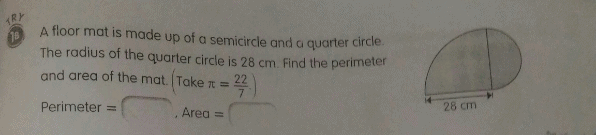# QuestionHi can anyone help me this question? Thanks

perimeter = circumstance of quadrant + semicircle + 28cm

= 1/4(2 * 22/7 * 28) + 1/2(2* 22/7 * 14) + 28

= 116cm

area = 1/4 (22/7 * 28 * 28) + 1/2 (22/7 * 14 * 14)

= 924 cm^2

0 Replies 0 Likes

Diameter of quadrant = 2 x 28cm = 56cm

Circumference of quadrant = 1/4 x 22/7 x 56cm = 44cm

Circumference of semicircle = 1/2 x 22/7 x 28cm = 44cm

Perimeter of figure = 28cm + 44cm + 44m = 116cm

Area of quadrant = 1/4 x 22/7 x 28cm x 28cm = 616cm2

Radius of semicircle = 28cm ÷ 2 = 14cm

Area of semicircle = 1/2 x 22/7 x 14cm x 14cm = 308cm2

Area of figure = 616cm2 + 308cm2 = 924cm2

Hope you understand!

0 Replies 1 Like